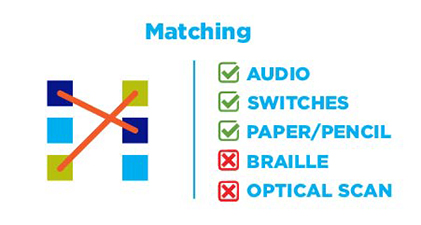# Matching

Matching labeling items require users to select a label in the first column for each element in the second column by drawing a line between the two columns. Matching items must be hand scored.1. Select the x value that makes each equation true.
2.  x = -3 x = -2 x​ = -4 x = -1 x2 – x – 6 = 0 3x2 – 12x – 15 = 0 6x2 – 6x – 72 = 0 6x2 + 18x – 24 = 0